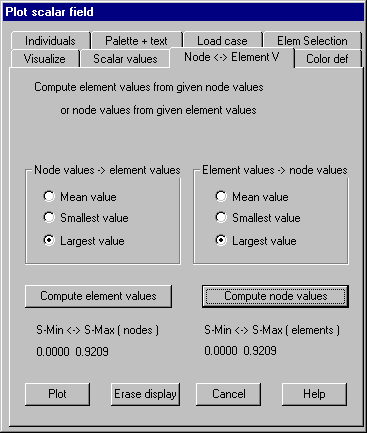Node « Element values: Conversion between node and element related scalar values

Depending on the kind of the visualization node or element related scalar values are needed. This property page defines how node related values are calculated when element related values are given and conversely.

If scalar values are given at the integration points of the elements, values for the element nodes and for additional nodes used for element approximation are calculated by interpolation respectively extrapolation using all given scalar values. If only one scalar value for the element is needed (for example plot of columns or plot with constant element color) a mean value is calculated using the factors of gauss integration.

Following dialog shows the available options:Node values ® Element values

Mean value: The mean of all values given at the nodes of the element is used for this element.

Smallest value: The smallest of all values given at the nodes is used.

Largest value: The largest of all values given at the nodes is used.

Element values ® Node values

Mean value: For each node of the structure the mean of the scalar values from all elements belonging to this node is used.

Smallest value: The smallest value from all associated elements is used.

Largest value: The largest value from all associated elements is use.

Compute element values

Clicking his button the scalar values for the elements are newly computed (available only if node values are given).

Compute node values

Clicking this button the scalar values for the nodes are newly computed (available only if element values are given).